# 4.4 Understand slope of a line  (Page 2/14)

 Page 2 / 14

What is the slope of the line on the geoboard shown?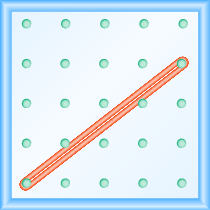## Solution

Use the definition of slope: $m=\frac{\text{rise}}{\text{run}}.$

Start at the left peg and count the spaces up and to the right to reach the second peg.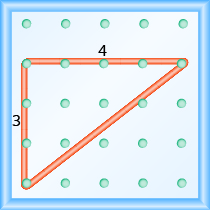$\begin{array}{ccc}\text{The rise is 3.}\hfill & & \phantom{\rule{5em}{0ex}}m=\frac{3}{\text{run}}\hfill \\ \text{The run is 4.}\hfill & & \phantom{\rule{5em}{0ex}}m=\frac{3}{4}\hfill \\ & & \phantom{\rule{5em}{0ex}}\text{The slope is}\phantom{\rule{0.4em}{0ex}}\frac{3}{4}.\hfill \end{array}$

This means that the line rises 3 units for every 4 units of run.

What is the slope of the line on the geoboard shown?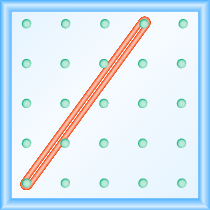$\frac{4}{3}$

What is the slope of the line on the geoboard shown?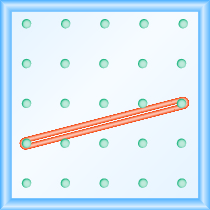$\frac{1}{4}$

What is the slope of the line on the geoboard shown?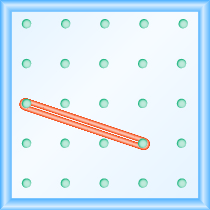## Solution

Use the definition of slope: $m=\frac{\text{rise}}{\text{run}}.$

Start at the left peg and count the units down and to the right to reach the second peg.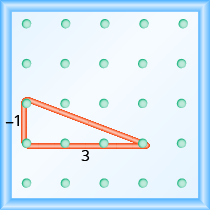$\begin{array}{cccc}\text{The rise is}\phantom{\rule{0.2em}{0ex}}-1.\hfill & & & \phantom{\rule{1.5em}{0ex}}m=\frac{-1}{\text{run}}\hfill \\ \text{The run is 3.}\hfill & & & \phantom{\rule{1.5em}{0ex}}m=\frac{-1}{3}\hfill \\ & & & \phantom{\rule{1.5em}{0ex}}m=-\frac{1}{3}\hfill \\ & & & \text{The slope is}\phantom{\rule{0.2em}{0ex}}-\frac{1}{3}.\hfill \end{array}$

This means that the line drops 1 unit for every 3 units of run.

What is the slope of the line on the geoboard?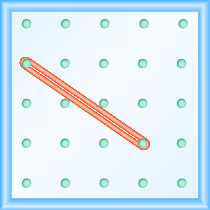$-\frac{2}{3}$

What is the slope of the line on the geoboard?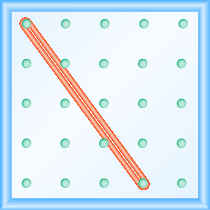$-\frac{4}{3}$

Notice that in [link] the slope is positive and in [link] the slope is negative. Do you notice any difference in the two lines shown in [link] (a) and [link] (b)?

We ‘read’ a line from left to right just like we read words in English. As you read from left to right, the line in [link] (a) is going up; it has positive slope    . The line in [link] (b) is going down; it has negative slope    .

## Positive and negative slopes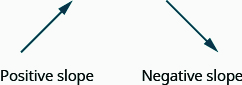Use a geoboard to model a line with slope $\frac{1}{2}$ .

## Solution

To model a line on a geoboard, we need the rise and the run.

$\begin{array}{ccccc}\text{Use the slope formula.}\hfill & & \hfill \phantom{\rule{8em}{0ex}}m& =\hfill & \frac{\text{rise}}{\text{run}}\hfill \\ \text{Replace}\phantom{\rule{0.2em}{0ex}}m\phantom{\rule{0.2em}{0ex}}\text{with}\phantom{\rule{0.2em}{0ex}}\frac{1}{2}.\hfill & & \hfill \phantom{\rule{8em}{0ex}}\frac{1}{2}& =\hfill & \frac{\text{rise}}{\text{run}}\hfill \end{array}$

So, the rise is 1 and the run is 2.

Start at a peg in the lower left of the geoboard.

Stretch the rubber band up 1 unit, and then right 2 units.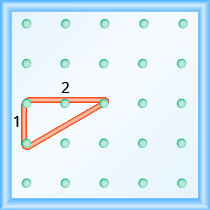The hypotenuse of the right triangle formed by the rubber band represents a line whose slope is $\frac{1}{2}$ .

Model the slope $m=\frac{1}{3}$ . Draw a picture to show your results.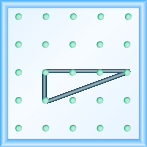Model the slope $m=\frac{3}{2}$ . Draw a picture to show your results.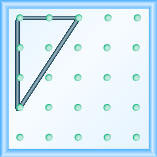Use a geoboard to model a line with slope $\frac{-1}{4}.$

## Solution

$\begin{array}{ccccc}\text{Use the slope formula.}\hfill & \phantom{\rule{9em}{0ex}}\hfill & \hfill m& =\hfill & \frac{\text{rise}}{\text{run}}\hfill \\ \text{Replace}\phantom{\rule{0.2em}{0ex}}m\phantom{\rule{0.2em}{0ex}}\text{with}\phantom{\rule{0.5em}{0ex}}\frac{-1}{\phantom{\rule{0.4em}{0ex}}4}.\hfill & \phantom{\rule{9em}{0ex}}\hfill & \hfill \frac{-1}{\phantom{\rule{0.4em}{0ex}}4}& =\hfill & \frac{\text{rise}}{\text{run}}\hfill \end{array}$

So, the rise is $-1$ and the run is 4.

Since the rise is negative, we choose a starting peg on the upper left that will give us room to count down.

We stretch the rubber band down 1 unit, then go to the right 4 units, as shown.The hypotenuse of the right triangle formed by the rubber band represents a line whose slope is $\frac{-1}{4}$ .

Model the slope $m=\frac{-2}{3}$ . Draw a picture to show your results.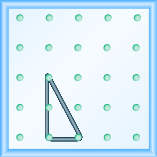Model the slope $m=\frac{-1}{3}$ . Draw a picture to show your results.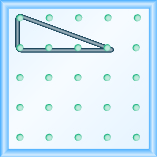## Use $m=\frac{\text{rise}}{\text{run}}$ To find the slope of a line from its graph

Now, we’ll look at some graphs on the $xy$ -coordinate plane and see how to find their slopes. The method will be very similar to what we just modeled on our geoboards.

To find the slope, we must count out the rise and the run. But where do we start?

We locate two points on the line whose coordinates are integers. We then start with the point on the left and sketch a right triangle, so we can count the rise and run.

## How to use $m=\frac{\text{rise}}{\text{run}}$ To find the slope of a line from its graph

Find the slope of the line shown.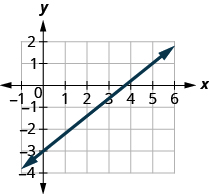## Solution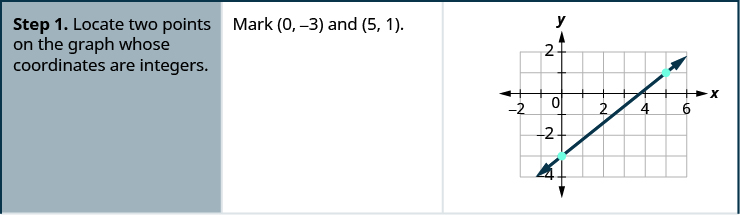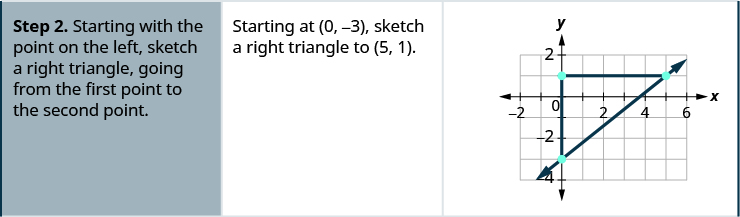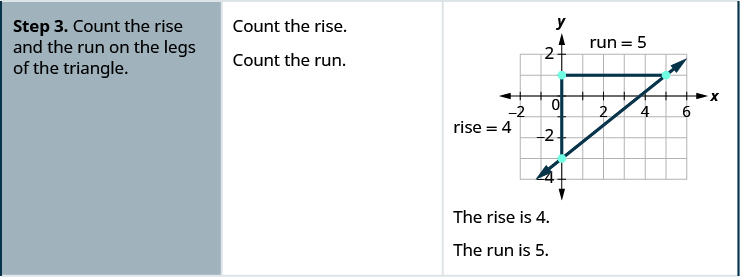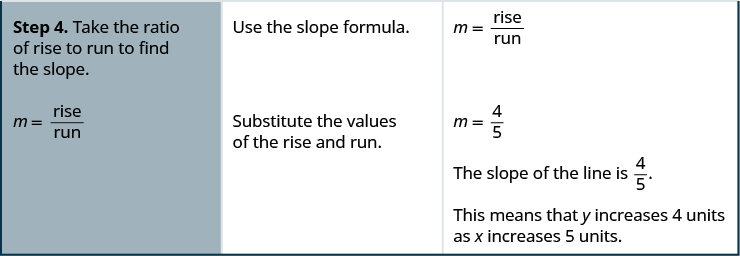Find the slope of the line shown.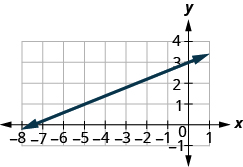$\frac{2}{5}$

Find the slope of the line shown.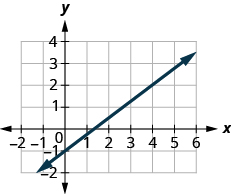$\frac{3}{4}$

## Find the slope of a line from its graph using $m=\frac{\text{rise}}{\text{run}}.$

1. Locate two points on the line whose coordinates are integers.
2. Starting with the point on the left, sketch a right triangle, going from the first point to the second point.
3. Count the rise and the run on the legs of the triangle.
4. Take the ratio of rise to run to find the slope, $m=\frac{\text{rise}}{\text{run}}$ .

Aziza is solving this equation-2(1+x)=4x+10
No. 3^32 -1 has exactly two divisors greater than 75 and less than 85 what is their product?
x^2+7x-19=0 has Two solutions A and B give your answer to 3 decimal places
3. When Jenna spent 10 minutes on the elliptical trainer and then did circuit training for20 minutes, her fitness app says she burned 278 calories. When she spent 20 minutes onthe elliptical trainer and 30 minutes circuit training she burned 473 calories. How manycalories does she burn for each minute on the elliptical trainer? How many calories doesshe burn for each minute of circuit training?
.473
Angelita
?
Angelita
John left his house in Irvine at 8:35 am to drive to a meeting in Los Angeles, 45 miles away. He arrived at the meeting at 9:50. At 3:30 pm, he left the meeting and drove home. He arrived home at 5:18.
p-2/3=5/6 how do I solve it with explanation pls
P=3/2
Vanarith
1/2p2-2/3p=5p/6
James
Cindy
4.5
Ruth
is y=7/5 a solution of 5y+3=10y-4
yes
James
Cindy
Lucinda has a pocketful of dimes and quarters with a value of $6.20. The number of dimes is 18 more than 3 times the number of quarters. How many dimes and how many quarters does Lucinda have? Rhonda Reply Find an equation for the line that passes through the point P ( 0 , − 4 ) and has a slope 8/9 . Gabriel Reply is that a negative 4 or positive 4? Felix y = mx + b Felix if negative -4, then -4=8/9(0) + b Felix -4=b Felix if positive 4, then 4=b Felix then plug in y=8/9x - 4 or y=8/9x+4 Felix Macario is making 12 pounds of nut mixture with macadamia nuts and almonds. macadamia nuts cost$9 per pound and almonds cost $5.25 per pound. how many pounds of macadamia nuts and how many pounds of almonds should macario use for the mixture to cost$6.50 per pound to make?
Nga and Lauren bought a chest at a flea market for $50. They re-finished it and then added a 350 % mark - up Makaila Reply$1750
Cindy
the sum of two Numbers is 19 and their difference is 15
2, 17
Jose
interesting
saw
4,2
Cindy
Felecia left her home to visit her daughter, driving 45mph. Her husband waited for the dog sitter to arrive and left home 20 minutes, or 13 hour later. He drove 55mph to catch up to Felecia. How long before he reaches her?
hola saben como aser un valor de la expresión
NAILEA
integer greater than 2 and less than 12
2 < x < 12
Felix
I'm guessing you are doing inequalities...
Felix
Actually, translating words into algebraic expressions / equations...
Felix
hi
Darianna
hello
Mister
Eric here
Eric
6
Cindy

#### Get Jobilize Job Search Mobile App in your pocket Now!By OpenStaxBy Zarina ChocolateByBy Rebecca ButterfieldBy Samuel MaddenBy JavaChamp TeamBy Stephen VoronBy Richley CrapoBy OpenStaxBy Danielle Stephens